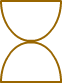# Rotational Symmetry

Print
Rate 0 stars
Common Core
• Lesson size:
Message preview:
Someone you know has shared lesson with you:

To play this lesson, click on the link below:

https://www.turtlediary.com/lesson/rotational-symmetry.html

Hope you have a good experience with this site and recommend to your friends too.

Login to rate activities and track progress.
Login to rate activities and track progress.

A figure has rotational symmetry if you can rotate (turn) it and it would still look the same.

For instance:You can turn the star, shown in the above figure, in 5 different ways and it will look exactly the same.

Imagine, if you had two of these stars positioned on the top of each other and you could flip the one on to the other, they will appear as one.

Lets take another example:

## Example 1In this example, the triangle does not have rotational symmetry. If you rotate it, it will not look exactly the same.

Lets consider some examples of rotational symmetry:

## Example 1This flower does have rotational symmetry because it could be turned and would look exactly the same. It could be turned 5 times, so the order is 5.

## Example 2This figure does have rotational symmetry because it could be turned 180°, and would look exactly the same.

The order is only 2, though, because it could only be in 2 positions: the one it is now and the other is upside down position.

Remember that whenever a figure has order 2 rotational symmetry, it has point symmetry (or origin symmetry.) That's because each corresponding point is the same distance from the center point but in opposite directions.

## Example 3This stick figure does not have rotational symmetry. If you turn him, he will not look the same as

he does now. (He'll be crooked, sideways, or in upside down position.)

## Example 4This figure does have rotational symmetry because it could be turned and would look exactly the same. It could be turned 3 times, so the order is 3.

### Fun fact: The word "symmetry" comes from the Greek words 'syn' (together) and 'metron' (measure).

If a shape looks exactly the same after one full rotation, there is really no symmetry at all.

The order of rotational symmetry is the number of positions you can turn the object and still have it look exactly the same. If it looks the same in two different positions, it is order 2; if it looks the same in 3 different positions, it is order 3, and so on.

Point symmetry (or origin symmetry) occurs whenever a figure has order 2 rotational symmetry. This means that each point on the object has a corresponding point that is the exact same distance from the center point but in the opposite direction.

## Rotational Symmetry

• A figure has rotational symmetry if you can rotate (turn) it and it would still look the same.
• The order of rotational symmetry is found by determining how many ways you can turn the object and still have it look exactly the same.

## Similar Lessons

Become premium member to get unlimited access.